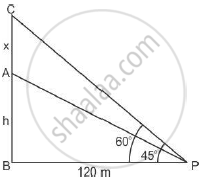# The angle of elevation of the top of a tower at a distance of 120 m from a point A on the ground is 45°. If the angle of elevation of the top of a flagstaff fixed at the top of the tower, at A is 60°, then find the height of the flagstaff. - Mathematics

The angle of elevation of the top of a tower at a distance of 120 m from a point A on the ground is 45°. If the angle of elevation of the top of a flagstaff fixed at the top of the tower, at A is 60°, then find the height of the flagstaff. [use √3=1.73]

#### Solution

Solution:
Let AB is the tower of height h meter and AC is flagstaff of height x meter.∠APB=45° and ∠BPC =60°

Tan 60 = (x+h)/120

sqrt3=(x+h)/120

x=120sqrt3-h

tan 45=h/120

therefore height of the flagstaff =

=120sqrt3-120

=120(sqrt3-1)

=120 xx73

=87.6 cm

Concept: Heights and Distances
Is there an error in this question or solution?